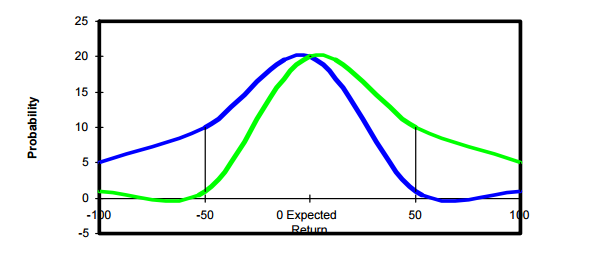### Standard Deviation is a Poor Measure of Risk

John Koch Senior Investment Analyst |
Categories

Insights and observations into the psychology of investing can enhance the understanding and measure of risk. A core innovation of the iSectors® Post-MPT Allocations is their recognition that standard deviation is a poor proxy for how human beings experience risk. Risk is an emotional condition: fear of a bad outcome such as fear of loss, fear of underperformance, or fear of failing to achieve a financial goal. iSectors® Post-MPT’s asset allocation models manage risk by setting a zero tolerance of going below zero, rather than measuring risk using standard deviation.  This approach also deals with non-symmetric (skewed) return distributions. Managing risk using a threshold of zero recognizes that losing money is the risk investors want to reduce.

Below is an illustrated example of how standard deviation is a poor measure of risk, specifically within the mean-variance optimization framework.If we were to analyze each of the investments represented by the green frequency of returns curve and the blue frequency of returns curve using mean-variance optimization (MVO) and standard deviation (SD), we’d consider them both equal. Both these investments have the same expected return and standard deviation.

Both investments have the same SD because they are equally volatile. That is, they both deviate from the expected return equally. Yet these are not equal investments: green’s volatility is upside and blue’s volatility is downside.

Using SD as a measure of risk under MVO, we would have overlooked a great investment simply because it had unexpectedly positive upside returns.  This happens because when using standard deviation, upside volatility gets penalized the same as downside volatility. The idea that unexpected gain is as risky as unexpected loss shows the limits of the theoretical construct to appropriate what risk really means to the real-life investor.

Standard deviation as a measure of risk is not consistent with the way the concept of risk actually operates when an investor makes investment decisions. To the degree that MVO assumes risk can be measured by standard deviation, MVO will fail to account for the psychological or behavioral aspects of investor decision-making mentioned earlier. A portfolio optimization formula that incorporates an investor’s concerns about losing money would be more appropriate than MVO’s use of standard deviation as a measure of risk, which is exactly what the iSectors® Post-MPT Allocations do.